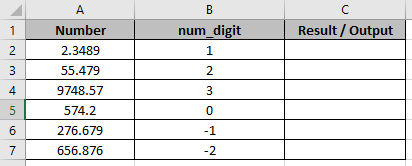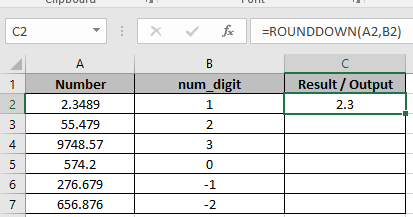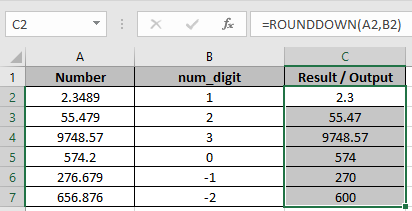# How to Use Rounddown Function in Excel

In this article, we will learn how to use ROUNDOWN function in Excel.

ROUNDDOWN function Round downs the numbers from 1-9. Unlike Round function, round down the numbers less than 5. It takes 2 arguments

1. The Number and
2. Up to digit, we need to round up.

Syntax:

=ROUNDDOWN(number, num_digit)

Let’s use them in some examples to see how it works.In one Column there are Numbers and in other, there are num_digits up to which the number to be rounded down.
Use the formula in C2 cell.

=ROUNDDOWN(A2,B2)

Explanation:
Here in C2 cell, the number will be round off to 1 decimal place.As you can see 2.4 is rounded DOWN to 1 decimal place.
Copy the formula using Ctrl + D taking the first cell till the last cell needed formula.As you can see

1. First 3 cells are rounded down upto 1, 2 and 3 decimal places respectively.
2. In C5, The number is rounded down to the nearest whole number.
3. The last 2 cells are rounded down to the nearest 10 and 100 respectively.
To get the number rounded down upto the nearest whole number, 0 is used default as num_digit

Hope you understood how to use ROUNDDOWN function in Excel. Explore more articles on ROUND and ROUNDUP function here. State your queries in the comment box below.

Related Articles:

Formula Round to nearest 100 and 1000

How to Use ROUND function in Excel

How to Use RoundUp function in Excel

Rounding Numerical Calculation Results In Microsoft Excel

Popular Articles:

How to use the VLOOKUP Function in Excel

How to use the COUNTIF in Excel 2016

How to Use SUMIF Function in Excel

Terms and Conditions of use

The applications/code on this site are distributed as is and without warranties or liability. In no event shall the owner of the copyrights, or the authors of the applications/code be liable for any loss of profit, any problems or any damage resulting from the use or evaluation of the applications/code.